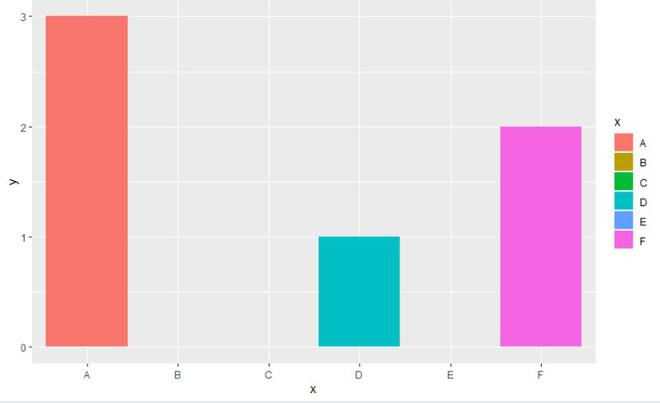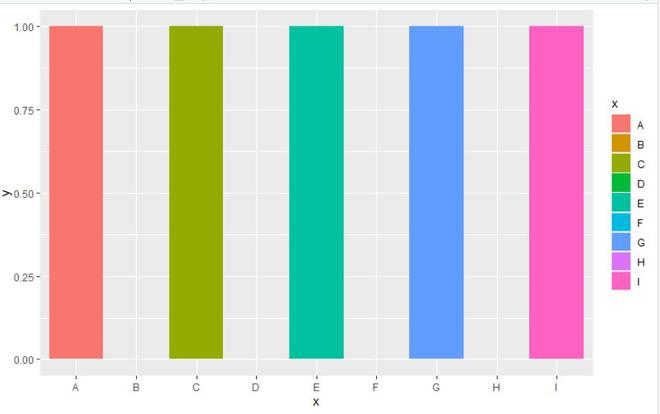# Keep Unused Factor Levels in ggplot2 Barplot in R

• Last Updated : 29 Jun, 2021

In this article, we will discuss how to keep unused factor levels in ggplot2 barplot in the R programming language.

In this approach to keep unused factor levels in ggplot2 barplot, the user first needs to install and import the ggplot2 package in the R console and plot the barplot of the data consisting of zero values with the geom_bar() and ggplot() function called with the required parameters and then call the scale_x_discrete() function with the drop argument to be set as false form the ggplot2 package to keep unused factor levels in the R programming language.

Syntax:

scale_x_discrete(…, expand = waiver())

Parameter:

• …: common discrete scale parameters: name, breaks, labels, na.value, limits and guide.
• expand: a numeric vector of length two giving multiplicative and additive expansion constants. These constants ensure that the data is placed some distance away from the axes.

Example 1:

## R

 `library``(``"ggplot2"``) ``gfg <- ``data.frame``(x = ``c``(``'A'``,``'B'``,``'C'``,``'D'``,``'E'``,``'F'``),        ``                   ``y = ``c``(3, 0, 0, 1, 0,2))``                            ` `ggp <- ``ggplot``(gfg, ``aes``(x, y, fill = x)) +  ``geom_bar``(stat = ``"identity"``)``          ` `ggp +  ``scale_x_discrete``(drop = ``FALSE``)`` ` `ggp`

Output:Example 2:

## R

 `library``(``"ggplot2"``) `` ` ` ` `gfg <- ``data.frame``(x = ``c``(``'A'``,``'B'``,``'C'``,``'D'``,``'E'``,``'F'``,``'G'``,``'H'``,``'I'``),        ``                  ``y = ``c``(1,0,1,0,1,0,1,0,1))`` ` `ggp <- ``ggplot``(gfg, ``aes``(x, y, fill = x)) +  ``geom_bar``(stat = ``"identity"``)`` ` `ggp +  ``scale_x_discrete``(drop = ``FALSE``)``   ` `ggp`

Output:My Personal Notes arrow_drop_up